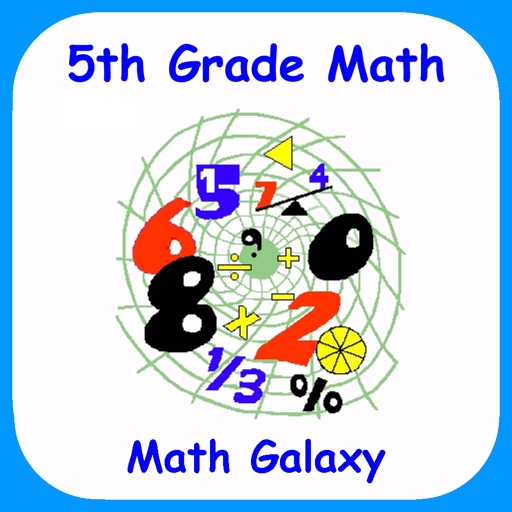"5th Grade Math by Math Galaxy is a comprehensive review and practice app covering all of the basics for the grade level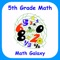5th Grade Math - Math Galaxy

by Math Galaxy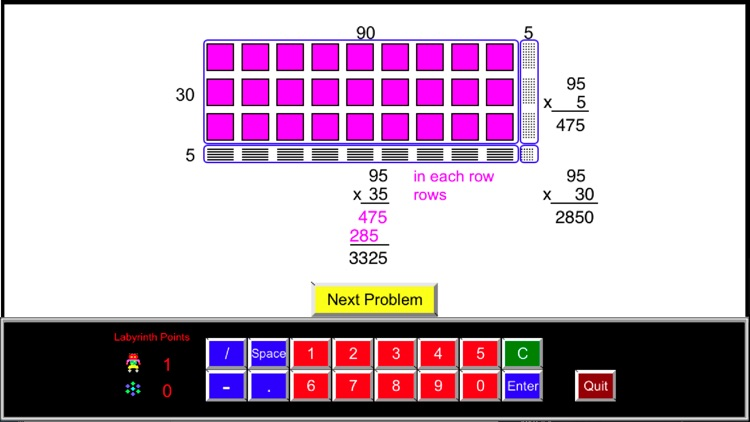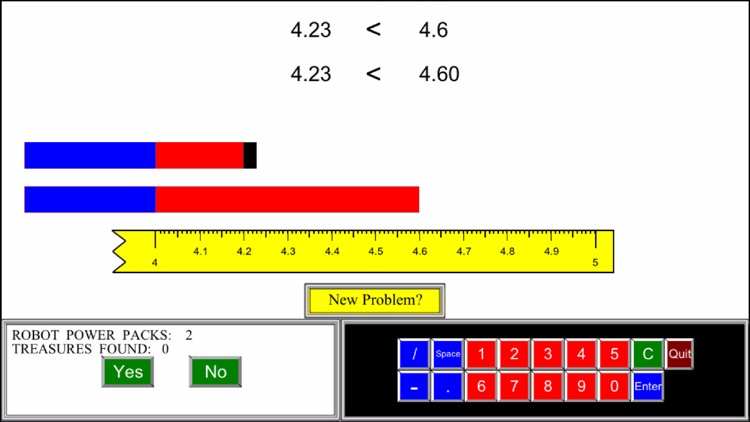"5th Grade Math by Math Galaxy is a comprehensive review and practice app covering all of the basics for the grade level. This easy to navigate app offers kids a great way to get that extra little bit of help that they might need to solidify their understanding of basic math concepts."Version
3.1
Rating
(9)
Size
187Mb
Genre
Last updated
April 13, 2019
Release date
October 11, 2014

App Screenshots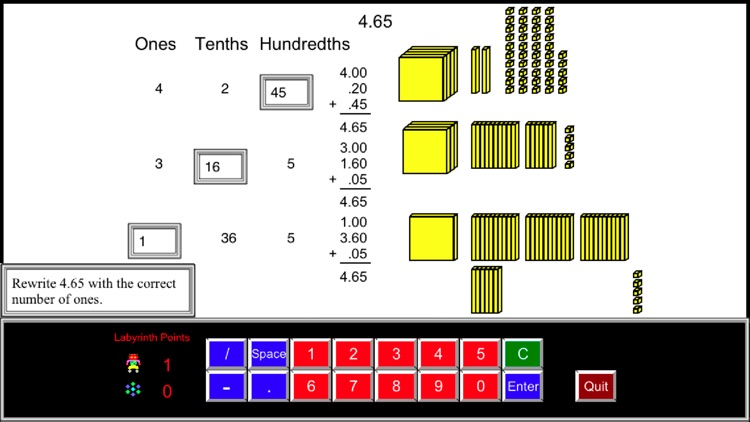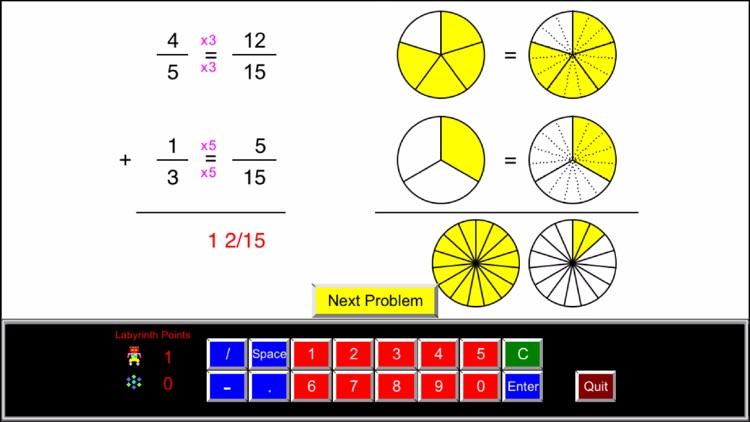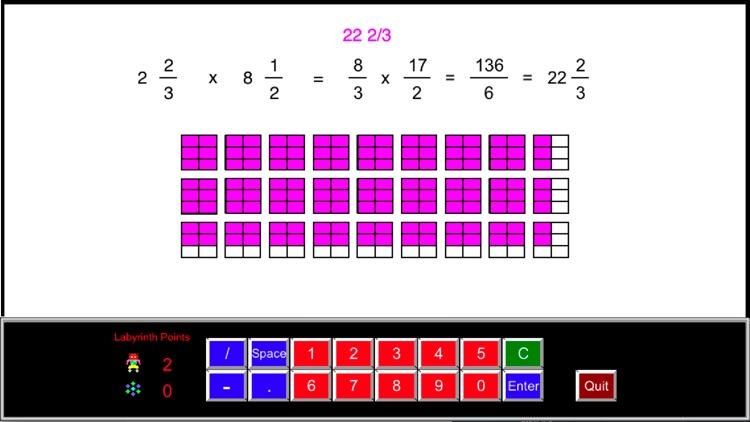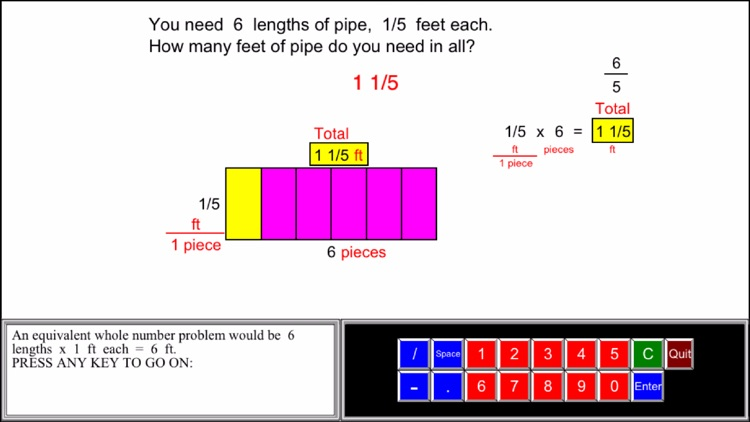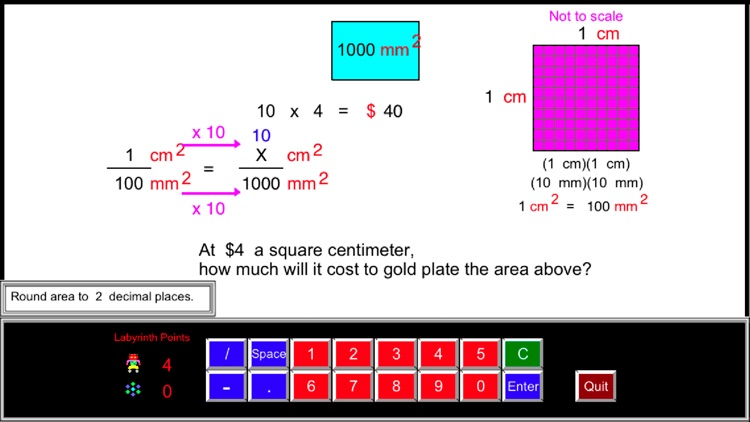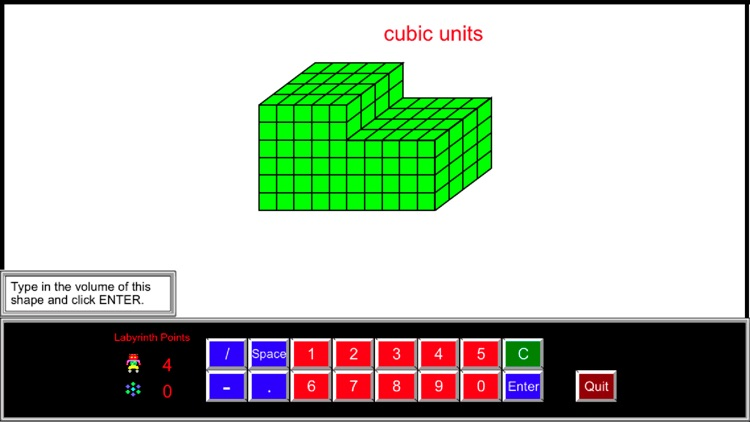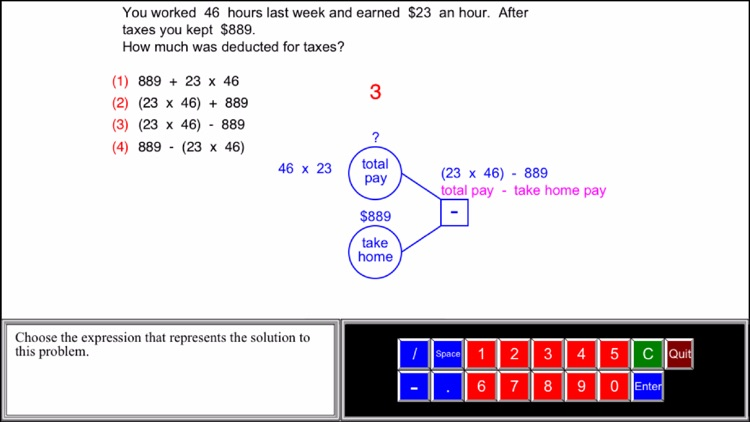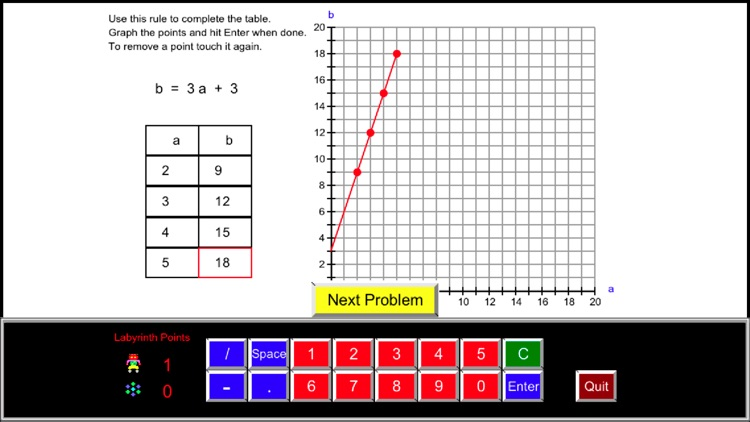App Store Description

"5th Grade Math by Math Galaxy is a comprehensive review and practice app covering all of the basics for the grade level. This easy to navigate app offers kids a great way to get that extra little bit of help that they might need to solidify their understanding of basic math concepts."

"We have been able to find each and every math concept that we have been presented with this year outlined neatly within this app."

See the rest of the review at:

In fifth grade math the emphasis is on fractions, decimals and volume: (1) developing fluency with addition and subtraction of fractions and multiplication and division of fractions and whole numbers, (2) decimal operations to hundredths and whole number division with 2-digit divisors, and (3) volume.

Math Galaxy’s Fifth Grade Math covers all of the Common Core math standards for fifth grade as listed below.
The concepts are presented in bite-size pieces with an unlimited supply of practice problems, with numbers chosen randomly.
Illustrations are used throughout to reinforce the concepts.
By solving problems students earn robots to use in a maze game, plus there are several other games involving math practice.
Certificates and summaries of scores can be emailed to be printed.

NUMBERS AND OPERATIONS IN BASE 10 (5.NBT)

Understand the place value system.
Perform operations with multi-digit whole numbers, and with decimals to hundredths.

Place Value (5.NBT.1, 5.NBT.3a)
Place Names
Expanded Notation

Regrouping (5.NBT.1)
(Whole #s & Decimals)

Comparing Decimals (5.NBT.3b)

Rounding Decimals (5.NBT.4)

Multiplying & Dividing by 10s (5.NBT.2)
Multiply and Divide Taking Out Factors of 10 (5.NBT.1,2)

Multiplying Whole Numbers (5.NBT.5)

Dividing Whole Numbers (5.NBT.6)
(2-3 Digit Divisors, Traditional & Alternative)

Decimal Operations (5.NBT.7)
Subtracting
Multiplying
Dividing
Word Problems

FRACTIONS (5.NF)

Use equivalent fractions as a strategy to add and subtract fractions.
Apply and extend previous understandings of multiplication and division to multiply and divide fractions.

Multiplying Fractions (5.NF.4)
Fractions as Scaling (5.NF.5)
Dividing Unit Fractions (5.NF.7)
Dividing Simple Fractions (5.NF.7)
Dividing Mixed Numbers (5.NF.7)
Fraction Word Problems (5.NF.2, 6, 7c)
Fractions as Division (5.NF.3)
Multiply or Divide
All Operations

MEASUREMENT AND DATA (5.MD)

Convert like measurement units within a given measurement system.
Understand concepts of volume and relate volume to multiplication and addition.

Length (5.MD.1)
Area (5.MD.1)
Mass (5.MD.1)
Volume
Converting Units (5.MD.1)
Cubic Units 1 (5.MD.3, 5.MD.4)
Cubic Units 2 (5.MD.5c)
Missing Side (5.MD.5a, 5b)
Line Plots (5.MD.2)

GEOMETRY (5.G)

Graph points on the coordinate plane.
Classify two-dimensional figures into categories based on their properties.

Graphing Coordinates (5.G1, 5.G2)
Lines, Angles, & Polygons (5.G.3, 5.G.4)

ALGEBRAIC THINKING (5.OA)

Write and interpret numerical expressions.
Analyze patterns and relationships.

Algebraic Operations (5.OA.1, 5.OA.2)
Translating to Algebra (5.OA.2)
Order of Operations (5.OA..1)
Algebraic Patterns (5.OA.3)

GAMES

Riddles (Length, Area, Volume, & Time - in Measurement section)
Labyrinth (Guide robots through mazes in search of treasures.
Robots are earned by solving problems.)
The Money Trail
Bridge the Swamp (adding, subtracting, multiplying, & dividing fractions and decimals)

Disclaimer:
AppAdvice does not own this application and only provides images and links contained in the iTunes Search API, to help our users find the best apps to download. If you are the developer of this app and would like your information removed, please send a request to [email protected] and your information will be removed.